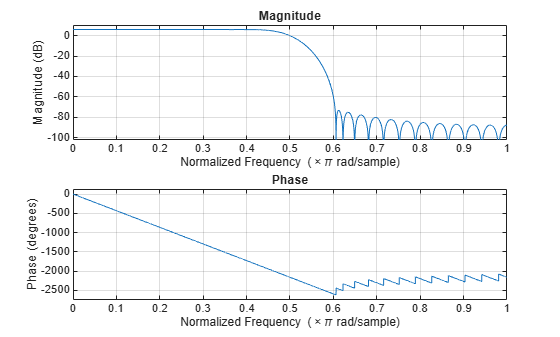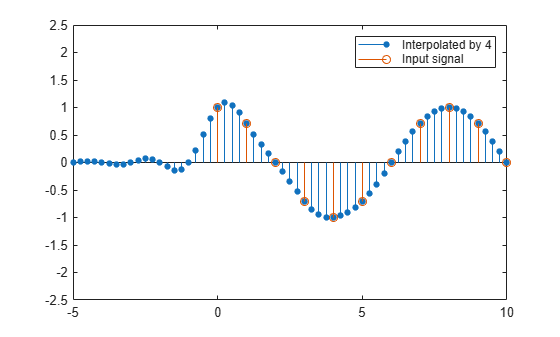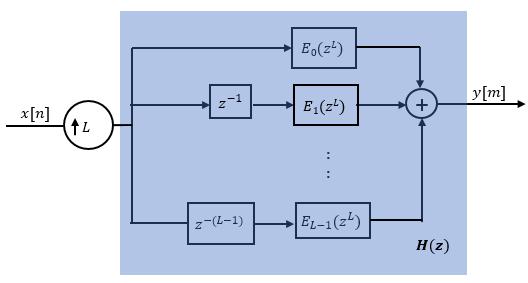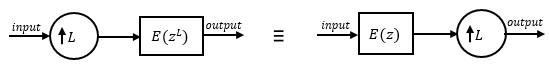# dsp.FIRInterpolator

Polyphase FIR interpolator

## Description

The `dsp.FIRInterpolator` System object™ upsamples an input by the integer upsampling factor L along the first dimension. The FIR interpolator (as shown in the schematic) conceptually consists of an upsampler followed by an FIR anti-imaging filter, which is usually an approximation of an ideal band-limited interpolation filter. The coefficients of the anti-imaging filter can be specified through the `Numerator` property, or can be automatically designed by the object using the `designMultirateFIR` function.

The upsampler upsamples each channel of the input to a higher rate by inserting L–1 zeros between samples. The FIR filter that follows filters each channel of the upsampled data. The resulting discrete-time signal has a sample rate that is L times the original sample rate.Note that the actual object algorithm implements a direct-form FIR polyphase structure, an efficient equivalent of the combined system depicted in the diagram. For more details, see Algorithms.

To upsample an input:

1. Create the `dsp.FIRInterpolator` object and set its properties.

2. Call the object with arguments, as if it were a function.

Under specific conditions, this System object also supports SIMD code generation. For details, see Code Generation.

## Creation

### Syntax

``firinterp = dsp.FIRInterpolator``
``firinterp = dsp.FIRInterpolator(L)``
``firinterp = dsp.FIRInterpolator(L,'Auto')``
``firinterp = dsp.FIRInterpolator(L,num)``
``firinterp = dsp.FIRInterpolator(L,method)``
``firinterp = dsp.FIRInterpolator(___,Name,Value)``
``firinterp = dsp.FIRInterpolator(L,'legacy')``

### Description

````firinterp = dsp.FIRInterpolator` returns an FIR interpolator with an interpolation factor of 3. The object designs the FIR filter coefficients using the `designMultirateFIR(3,1)` function.```
````firinterp = dsp.FIRInterpolator(L)` returns an FIR interpolator with the integer-valued `InterpolationFactor` property set to `L`. The object designs its filter coefficients based on the interpolation factor `L` that you specify while creating the object using the `designMultirateFIR(L,1)` function. The designed filter corresponds to a lowpass with a cutoff at π/`L` in radial frequency units.```

example

````firinterp = dsp.FIRInterpolator(L,'Auto')` returns an FIR interpolator with the `NumeratorSource` property set to `'Auto'`. In this mode, every time there is an update in the interpolation factor, the object redesigns the filter using the design method specified in `DesignMethod`.```

example

````firinterp = dsp.FIRInterpolator(L,num)` returns an FIR interpolator with the `InterpolationFactor` property set to `L` and the `Numerator` property set to `num`.```
````firinterp = dsp.FIRInterpolator(L,method)` returns an FIR interpolator with the `InterpolationFactor` property set to `L` and the `DesignMethod` property set to `method`. When you pass the design method as an input, the `NumeratorSource` property is automatically set to `'Auto'`.```
````firinterp = dsp.FIRInterpolator(___,Name,Value)` returns an FIR interpolator object with each specified property set to the specified value. Enclose each property name in quotes. You can use this syntax with any previous input argument combinations.```
````firinterp = dsp.FIRInterpolator(L,'legacy')` returns an FIR interpolator where the filter coefficients are designed using `fir1(15,0.25)`. The designed filter has a cutoff frequency of 0.25π radians/sample.```

## Properties

expand all

Unless otherwise indicated, properties are nontunable, which means you cannot change their values after calling the object. Objects lock when you call them, and the `release` function unlocks them.

If a property is tunable, you can change its value at any time.

Specify the integer factor, L, by which to increase the sampling rate of the input signal. The polyphase implementation uses L polyphase subfilters to compute convolutions at the lower sample rate. The FIR interpolator delays and interleaves these lower-rate convolutions to obtain the higher-rate output.

Data Types: `single` | `double` | `int8` | `int16` | `int32` | `int64` | `uint8` | `uint16` | `uint32` | `uint64`

FIR filter coefficient source, specified as either:

• `'Property'` –– The numerator coefficients are specified through the `Numerator` property.

• `'Input port'` –– The numerator coefficients are specified as an input to the object algorithm.

• `'Auto'` –– The numerator coefficients are designed automatically using the design method specified in `DesignMethod`.

Numerator coefficients of the anti-imaging FIR filter, specified as a row vector in powers of z–1. The following equation defines the system function for a filter of length N+1:

`$H\left(z\right)=\sum _{n=0}^{N}b\left(n\right){z}^{-n}$`

The vector b = [b(0), b(1), …, b(N)] represents the vector of filter coefficients.

To act as an effective anti-imaging filter, the coefficients usually correspond to a lowpass filter with a normalized cutoff frequency no greater than the reciprocal of the `InterpolationFactor`. Use `designMultirateFIR` to design such a filter. More generally, any complex bandpass filter can be used. For an example, see Double the Sample Rate Using FIR Interpolator.

The filter coefficients are scaled by the value of the `InterpolationFactor` property before filtering the signal. To form the L polyphase subfilters, `Numerator` is appended with zeros if necessary.

#### Dependencies

This property is visible only when you set `NumeratorSource` to `'Property'`.

When `NumeratorSource` is set to `'Auto'`, the numerator coefficients are automatically redesigned using the design method specified in `DesignMethod`. To access the filter coefficients in the automatic design mode, type `objName.``Numerator` in the MATLAB® command prompt.

Data Types: `single` | `double` | `int8` | `int16` | `int32` | `int64` | `uint8` | `uint16` | `uint32` | `uint64`
Complex Number Support: Yes

Design method of the FIR filter coefficients, specified as one of the following:

• `'Kaiser'` –– Kaiser method. Approximate anti-imaging lowpass filter using the `designMultirateFIR` function.

• `'ZOH'` –– Zero order hold method. Hold the input sequence values.

• `'Linear'` –– Linear interpolation method.

#### Dependencies

This property is visible only when you set the `NumeratorSource` property to `'Auto'`, or if you pass the `'auto'` keyword as an input while creating the object.

### Fixed-Point Properties

Flag to use full-precision rules for fixed-point arithmetic, specified as one of the following:

• `true` –– The object computes all internal arithmetic and output data types using the full-precision rules. These rules provide the most accurate fixed-point numerics. In this mode, other fixed-point properties do not apply. No quantization occurs within the object. Bits are added, as needed, to ensure that no roundoff or overflow occurs.

• `false` –– Fixed-point data types are controlled through individual fixed-point property settings.

For more information, see Full Precision for Fixed-Point System Objects and Set System Object Fixed-Point Properties.

Rounding method for fixed-point operations. For more details, see rounding mode.

#### Dependencies

This property is not visible and has no effect on the numerical results when the following conditions are met:

• `FullPrecisionOverride` set to `true`.

• `FullPrecisionOverride` set to `false`, `ProductDataType` set to `'Full precision'`, `AccumulatorDataType` set to ```'Full precision'```, and `OutputDataType` set to `'Same as accumulator'`.

Under these conditions, the object operates in full precision mode.

Overflow action for fixed-point operations, specified as one of the following:

• `'Wrap'` –– The object wraps the result of its fixed-point operations.

• `'Saturate'` –– The object saturates the result of its fixed-point operations.

For more details on overflow actions, see overflow mode for fixed-point operations.

#### Dependencies

This property is not visible and has no effect on the numerical results when the following conditions are met:

• `FullPrecisionOverride` set to `true`.

• `FullPrecisionOverride` set to `false`, `OutputDataType` set to `'Same as accumulator'`, `ProductDataType` set to ```'Full precision'```, and `AccumulatorDataType` set to `'Full precision'`

Under these conditions, the object operates in full precision mode.

Data type of the FIR filter coefficients, specified as:

• `Same word length as input` –– The word length of the coefficients is the same as that of the input. The fraction length is computed to give the best possible precision.

• `Custom` –– The coefficients data type is specified as a custom numeric type through the `CustomCoefficientsDataType` property.

Word and fraction lengths of the coefficients data type, specified as an autosigned `numerictype` (Fixed-Point Designer) with a word length of 16 and a fraction length of 15.

#### Dependencies

This property applies when you set the `CoefficientsDataType` property to `Custom`.

Data type of the product output in this object, specified as one of the following:

• `'Full precision'` –– The product output data type has full precision.

• `'Same as input'` –– The object specifies the product output data type to be the same as that of the input data type.

• `'Custom'` –– The product output data type is specified as a custom numeric type through the `CustomProductDataType` property.

For more information on the product output data type, see Multiplication Data Types.

#### Dependencies

This property applies when you set `FullPrecisionOverride` to `false`.

Word and fraction lengths of the product data type, specified as an autosigned numeric type with a word length of 32 and a fraction length of 30.

#### Dependencies

This property applies only when you set `FullPrecisionOverride` to `false` and `ProductDataType` to `'Custom'`.

Data type of an accumulation operation in this object, specified as one of the following:

• `'Full precision'` –– The accumulation operation has full precision.

• `'Same as product'` –– The object specifies the accumulator data type to be the same as that of the product output data type.

• `'Same as input'` –– The object specifies the accumulator data type to be the same as that of the input data type.

• `'Custom'` –– The accumulator data type is specified as a custom numeric type through the `CustomAccumulatorDataType` property.

#### Dependencies

This property applies when you set `FullPrecisionOverride` to `false`.

Word and fraction lengths of the accumulator data type, specified as an autosigned numeric type with a word length of 32 and a fraction length of 30.

#### Dependencies

This property applies only when you set `FullPrecisionOverride` to `false` and `AccumulatorDataType` to `'Custom'`.

Data type of the object output, specified as one of the following:

• `'Same as accumulator'` –– The output data type is the same as that of the accumulator output data type.

• `'Same as input'` –– The output data type is the same as that of the input data type.

• `'Same as product'` –– The output data type is the same as that of the product output data type.

• `'Custom'` –– The output data type is specified as a custom numeric type through the `CustomOutputDataType` property.

#### Dependencies

This property applies when you set `FullPrecisionOverride` to `false`.

Word and fraction lengths of the output data type, specified as an autosigned numeric type with a word length of 16 and a fraction length of 15.

#### Dependencies

This property applies only when you set `FullPrecisionOverride` to `false` and `OutputDataType` to `'Custom'`.

## Usage

### Syntax

``y = firinterp(x)``
``y = firinterp(x,num)``

### Description

example

````y = firinterp(x)` interpolates the input signal `x` along the first dimension, and outputs the upsampled and filtered values, `y`.```
````y = firinterp(x,num)` uses the FIR filter, `num`, to interpolate the input signal. This configuration is valid only when the `'NumeratorSource'` property is set to `'Input port'`.```

### Input Arguments

expand all

Data input, specified as a vector or a matrix. A P-by-Q input matrix is treated as Q independent channels, and the System object interpolates each channel over the first dimension and generates a P*L-by-Q output matrix, where L is the interpolation factor.

This object supports variable-size input and does not support complex unsigned fixed-point inputs.

Data Types: `single` | `double` | `int8` | `int16` | `int32` | `int64` | `uint8` | `uint16` | `uint32` | `uint64` | `fi`
Complex Number Support: Yes

FIR filter coefficients, specified as a row vector.

#### Dependencies

This input is accepted only when the `'NumeratorSource'` property is set to `'Input port'`.

Data Types: `single` | `double` | `int8` | `int16` | `int32` | `int64` | `uint8` | `uint16` | `uint32` | `uint64` | `fi`
Complex Number Support: Yes

### Output Arguments

expand all

FIR interpolator output, returned as a vector or a matrix of size P*L-by-Q, where L is the interpolation factor.

Data Types: `single` | `double` | `int8` | `int16` | `int32` | `int64` | `uint8` | `uint16` | `uint32` | `uint64` | `fi`
Complex Number Support: Yes

## Object Functions

To use an object function, specify the System object as the first input argument. For example, to release system resources of a System object named `obj`, use this syntax:

`release(obj)`

expand all

 `freqz` Frequency response of discrete-time filter System object `fvtool` Visualize frequency response of DSP filters `info` Information about filter System object `cost` Estimate cost of implementing filter System object `polyphase` Polyphase decomposition of multirate filter `generatehdl` Generate HDL code for quantized DSP filter (requires Filter Design HDL Coder) `impz` Impulse response of discrete-time filter System object `coeffs` Returns the filter System object coefficients in a structure
 `step` Run System object algorithm `release` Release resources and allow changes to System object property values and input characteristics `reset` Reset internal states of System object

## Examples

collapse all

Interpolate a cosine wave by a factor of 2. In the automatic filter design mode, change the underlying D/A signal interpolation model to `'linear' `and interpolate the signal by a factor of 4, change the underlying D/A signal interpolation model to `'ZOH'` and interpolate the signal by a factor of 5.

The cosine wave has an angular frequency of $\frac{\pi }{4}$ radians/sample.

`x = cos(pi/4*(0:39)');`

Design Default Filter

Create a `dsp.FIRInterpolator` object. The object uses an anti-imaging lowpass filter after upsampling. By default, the anti-imaging lowpass filter is designed using the `designMultirateFIR` function. The function designs the filter based on the interpolation factor that you specify, and stores the coefficients in the `Numerator` property. For an interpolation factor of 2, the object designs the coefficients using `designMultirateFIR(2,1)`.

`firinterp = dsp.FIRInterpolator(2)`
```firinterp = dsp.FIRInterpolator with properties: InterpolationFactor: 2 NumeratorSource: 'Property' Numerator: [0 -2.0108e-04 0 7.7408e-04 0 -0.0020 0 0.0045 ... ] Show all properties ```

Visualize the filter response using `fvtool`. The designed filter meets the ideal filter constraints that are marked in red. The cutoff frequency is approximately half the spectrum.

`fvtool(firinterp)`Interpolate by 2

Interpolate the cosine signal by a factor of 2.

`y = firinterp(x);`

Plot the original and interpolated signals. In order to plot the two signals on the same plot, you must account for the output delay of the FIR interpolator and the scaling introduced by the filter. Use the `outputDelay` function to compute the `delay` value introduced by the interpolator. Shift the output by this delay value.

Visualize the input and the resampled signals. The input and output values coincide every other sample, due to the interpolation factor of 2.

`[delay,FsOut] = outputDelay(firinterp)`
```delay = 12 ```
```FsOut = 2 ```
```nx = (0:length(x)-1); ty = (0:length(y)-1)/FsOut-delay; stem(ty,y,'filled',MarkerSize=4); hold on; stem(nx,x); hold off; xlim([-5,20]) ylim([-2.5 2.5]) legend('Interpolated by 2','Input signal','Location','best');```Interpolate by 4 in Automatic Filter Design Mode

Now interpolate by a factor of 4. In order for the filter design to be updated automatically based on the new interpolation factor, set the `NumeratorSource` property to `'Auto'`. Alternately, you can pass the keyword `'Auto'` as an input while creating the object. The object then operates in the automatic filter design mode. Every time there is a change in the interpolation factor, the object updates the filter design.

```release(firinterp) firinterp.NumeratorSource = 'Auto'; firinterp.InterpolationFactor = 4```
```firinterp = dsp.FIRInterpolator with properties: InterpolationFactor: 4 NumeratorSource: 'Auto' DesignMethod: 'Kaiser' Show all properties ```

To access the filter coefficients in the automatic filter design mode, type `firinterp.Numerator` in the MATLAB command prompt.

The designed filter occupies a narrower passband that is approximately a quarter of the spectrum.

`fvtool(firinterp)`Interpolate the cosine signal by a factor of 4.

`yAuto = firinterp(x);`

Plot the original and resampled signals. Recalculate the delay and the output sample rate values since the interpolation factor has changed. The input and output values coincide every 4 output samples, owing to the interpolation factor of 4.

```[delay,FsOut] = outputDelay(firinterp); nx = (0:length(x)-1); tyAuto = (0:length(yAuto)-1)/FsOut-delay; stem(tyAuto,yAuto,'filled',MarkerSize=4); hold on; stem(nx,x); hold off; xlim([-5,10]) ylim([-2.5 2.5]) legend('Interpolated by 4','Input signal');```Specify Signal Interpolation Model

In the automatic design mode, you can also specify the underlying D/A signal interpolation model through the `DesignMethod` property.

Set `DesignMethod` to `'linear'`

If you set the `DesignMethod` to `'linear'`, the object uses the linear interpolation model.

```release(firinterp) firinterp.DesignMethod = 'linear'```
```firinterp = dsp.FIRInterpolator with properties: InterpolationFactor: 4 NumeratorSource: 'Auto' DesignMethod: 'Linear' Show all properties ```

Interpolate the signal using the linear interpolation model.

`ylinear = firinterp(x);`

Plot the original and the linearly interpolated signal.

```[delay,FsOut] = outputDelay(firinterp); nx = (0:length(x)-1); % Calculate output times for vector ylinear in input units tylinear = (0:length(ylinear)-1)/FsOut-delay; stem(tylinear,ylinear,'filled',MarkerSize=4); hold on; stem(nx,x); hold off; xlim([0,10]) ylim([-2.5 2.5]) legend('Linear Interpolation by 4','Input signal');```Set `DesignMethod` to `'ZOH'` and Change `InterpolationFactor` to 5

If you set the `DesignMethod` to `'ZOH'`, the object uses the zero order hold method. Change the interpolation factor to 5.

```release(firinterp) firinterp.DesignMethod = 'ZOH'; firinterp.InterpolationFactor = 5```
```firinterp = dsp.FIRInterpolator with properties: InterpolationFactor: 5 NumeratorSource: 'Auto' DesignMethod: 'ZOH' Show all properties ```

Interpolate the signal using the zero order hold method.

`yzoh = firinterp(x);`

Plot the original and ZOH interpolated signal.

```[delay,FsOut] = outputDelay(firinterp); nx = (0:length(x)-1); % Calculate output times for vector yzoh in input units tyzoh = (0:length(yzoh)-1)/FsOut-delay; stem(tyzoh,yzoh,'filled',MarkerSize=4); hold on; stem(nx,x); hold off; xlim([0,10]) ylim([-1.5 1.5]) legend('ZOH Interpolation by 4','Input signal');```Double the sample rate of an audio signal and play the interpolated signal using the `audioDeviceWriter` object.

Note: If you are using R2016a or an earlier release, replace each call to the object with the equivalent `step `syntax. For example, `obj(x) `becomes `step(obj,x)`.

Note: The `audioDeviceWriter` System object™ is not supported in MATLAB Online.

Create a `dsp.AudioFileReader` object. The default audio file ready by the object has a sample rate of 22050 Hz.

```afr = dsp.AudioFileReader('OutputDataType',... 'single');```

Create a `dsp.FIRInterpolator` object and specify the interpolation factor to be 2. The object designs the filter using the `designMultirateFIR(2,1)` function and stores the coefficients in the `Numerator` property of the object.

`firInterp = dsp.FIRInterpolator(2)`
```firInterp = dsp.FIRInterpolator with properties: InterpolationFactor: 2 NumeratorSource: 'Property' Numerator: [0 -2.0108e-04 0 7.7408e-04 0 -0.0020 0 0.0045 ... ] Show all properties ```

Create an `audioDeviceWriter` object. Specify the sample rate to be 22050$×$2, which equals 44100 Hz.

`adw = audioDeviceWriter(44100)`
```adw = audioDeviceWriter with properties: Device: 'Default' SampleRate: 44100 Show all properties ```

Read the audio signal using the file reader object, double the sample rate of the signal from 22050 Hz to 44100 Hz and play the interpolated signal.

```while ~isDone(afr) frame = afr(); y = firInterp(frame); adw(y); end pause(1); release(afr); release(adw);```

## Algorithms

The FIR interpolation filter is implemented efficiently using a polyphase structure.

To derive the polyphase structure, start with the transfer function of the FIR filter:

`$H\left(z\right)={b}_{0}+{b}_{1}{z}^{-1}+...+{b}_{N}{z}^{-N}$`

N+1 is the length of the FIR filter.

You can rearrange this equation as follows:

`$H\left(z\right)=\begin{array}{c}\left({b}_{0}+{b}_{L}{z}^{-L}+{b}_{2L}{z}^{-2L}+..+{b}_{N-L+1}{z}^{-\left(N-L+1\right)}\right)+\\ {z}^{-1}\left({b}_{1}+{b}_{L+1}{z}^{-L}+{b}_{2L+1}{z}^{-2L}+..+{b}_{N-L+2}{z}^{-\left(N-L+1\right)}\right)+\\ \begin{array}{c}⋮\\ {z}^{-\left(L-1\right)}\left({b}_{L-1}+{b}_{2L-1}{z}^{-L}+{b}_{3L-1}{z}^{-2L}+..+{b}_{N}{z}^{-\left(N-L+1\right)}\right)\end{array}\end{array}$`

L is the number of polyphase components, and its value equals the interpolation factor that you specify.

You can write this equation as:

`$H\left(z\right)={E}_{0}\left({z}^{L}\right)+{z}^{-1}{E}_{1}\left({z}^{L}\right)+...+{z}^{-\left(L-1\right)}{E}_{L-1}\left({z}^{L}\right)$`

E0(zL), E1(zL), ..., EL-1(zL) are polyphase components of the FIR filter H(z).

Conceptually, the FIR interpolation filter contains an upsampler followed by an FIR lowpass filter H(z).Replace H(z) with its polyphase representation.Here is the multirate noble identity for interpolation.Applying the noble identity for interpolation moves the upsampling operation to after the filtering operation. This move enables you to filter the signal at a lower rate.You can replace the upsampling operator, delay block, and adder with a commutator switch. The switch starts on the first branch 0 and moves in the counterclockwise direction, each time receiving one sample from each branch. The interpolator effectively outputs L samples for every one input sample it receives. Hence the sample rate at the output of the FIR interpolation filter is Lfs.## Version History

Introduced in R2012a